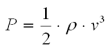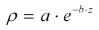# Air Density Calculator

Here you can calculated the air density either by using an aproximation for Switzerland or by using on-site measurements.

### Result

Air Density = 1.124 kg/m³

Calculated with the approximation for:
Altitude = 1000 m.ü.M.

### Approximation

 Altitude m

### Calculation

 Air Pressure hPa (500—1100 hPa) Temperature °C (-50°—50°C) relative humidity %

## Theory

The specific power P of the wind can be expressed by the following formula:where
ρ = air density
v = wind speed

From the equation it can be seen that the power of the wind and thereby the production of a wind turbine is proportional to air density. Colder air is denser than warmer air. Because air density decreases with height, it is importand — especially for a mountainous country such as Switzerland — to take air density into consideration in wind assessments for wind turbines.

### Approximation for Switzerland

The approximation for the air density ρ (for dry air) for Switzerland was established based in measurements from a number of meteorological stations:where
a = 1.247015
b = 0.000104
z = altitude [m]

Source: Swiss Federal Office of Energy, 1999: Planung von Windenergieanlagen - Leitfaden für die Schweiz

### Exact calculation of air density

The exact calculation of air density is done by measuring air pressure, temperature and humidity. These values can alternatively be obtained from nearby meteorological stations and extrapolated to the site. The respective equations can be found at the following link (in German):

» Wikipedia